# A Stock Trading Strategy With On-Balance Volume (OBV) & Python

Stock Trading Strategy Using On-Balance Volume (OBV) & Python

Before we begin, if you enjoy my articles and content and would like more content on programming, stocks, machine learning, etc. , then please give this article a few claps, it definitely helps out and I truly appreciate it ! So let’s begin !

In this article you will learn a simple trading strategy used to predict price movements using the Python programming language. More specifically you will learn how to perform algorithmic trading. It is extremely hard to try and predict the stock market momentum direction, but in this article I will give it a try. Even people with a good understanding of statistics and probabilities have a hard time doing this.

Algorithmic trading is a process for executing orders utilizing automated and pre-programmed trading instructions to account for variables such as price, timing and volume. -investopedia.com

Python is one of the hottest programming languages for finance, so we will use it and an indicator called the On-Balance Volume or (OBV) to create a trading strategy to know when to buy and sell stocks.

Disclaimer: The material in this article is purely educational and should not be taken as professional investment advice. Invest at your own discretion.

# What is On-Balance Volume (OBV)?

On-balance volume (OBV) is a technical trading momentum indicator that uses volume flow to predict changes in stock price.

Joseph Granville first developed the OBV metric in the 1963 book Granville’s New Key to Stock Market Profits.

Granville believed that volume was the key force behind markets and designed OBV to project when major moves in the markets would occur based on volume changes. In his book, he described the predictions generated by OBV as “a spring being wound tightly.” He believed that when volume increases sharply without a significant change in the stock’s price, the price will eventually jump upward or fall downward.-investopedia.com

OBV Trend Signals

• When both price and OBV are making higher peaks and higher troughs, the upward trend is likely to continue.
• When price continues to make lower troughs and OBV fails to make lower troughs, the downward trend is likely to stall or fail. This is called a positive divergence.
• During a trading range, if the OBV is rising, accumulation may be taking place — a warning of an upward breakout.
• During a trading range, if the OBV is falling, distribution may be taking place — a warning of a downward breakout.
• When a price continues to make higher peaks and the OBV fails to make higher peaks, the upward trend is likely to stall or fail. This is called a negative divergence.
• When price continues to make lower troughs and OBV fails to make lower troughs, the downward trend is likely to stall or fail. This is called a positive divergence.

You can also check out his book ‘How To Read The Stock Market’ which outlines his entire On-Balance Volume theory.

# How is On-Balance Volume (OBV) Calculated ?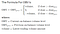Source
`If the closing price is above the prior close price then: Current OBV = Previous OBV + Current VolumeIf the closing price is below the prior close price then: Current OBV = Previous OBV  -  Current VolumeIf the closing prices equals the prior close price then:Current OBV = Previous OBV (no change)`

# When To Buy And Sell The Stock Using OBV ?

Traders that use OBV are interested in the rate of change of OBV to generate trade ideas. If the OBV is moving in the upward direction, it could give credence to the idea that a big move upward could be coming in price and vice versa if the OBV is moving in a downward direction, it could give credence to the idea that a big move downward could be coming in price.

For example if the OBV moves down faster than the corresponding move in price, then this could tell a trader that an even bigger move down in price is coming.

We will determine when to buy and sell stock by adding a moving average on the OBV, we’ll trade on crossover signals. This is the strategy that will be programmed in this article.

If OBV starts trading above the exponential moving average (EMA) then we will buy the stock.

If OBV starts trading below the exponential moving average (EMA) then we will sell the stock.

Note: Adding a 200-period exponential moving average to the OBV (a long-term moving average) will work better than a short-term moving average. The 200 EMA will produce fewer whipsaws. Whipsaw describes the movement of a security when, at a particular time, the security’s price is moving in one direction but then quickly pivots to move in the opposite direction.

If you prefer not to read this article and would like a video representation of it, you can check out the YouTube Video . It goes through everything in this article with a little more detail, and will help make it easy for you to start programming even if you don’t have the programming language Python installed on your computer. Or you can use both as supplementary materials for learning !

# KEY TAKEAWAYS

OBV is a simple running total of up day (added) and down day (subtracted) volume. If OBV is moving with price it confirms the current trend. Divergences between OBV and price indicates the price may be due for a reversal. Using trendlines can aid in spotting divergences and trading opportunities. OBV can also help forecast breakout directions in price. OBV is not without its faults though. Volume spikes can skew the indicator, making objective analysis more difficult. Also, while it may appear that OBV often leads price, this is often simply a case of searching for evidence we wish to find. Therefore, OBV is a tool to be used in addition to price analysis, but shouldn’t be relied on solely. -traderhq.com

• On-balance volume (OBV) is a technical indicator of momentum, using volume changes to make price predictions.
• OBV shows crowd sentiment that can predict a bullish or bearish outcome.
• Comparing relative action between price bars and OBV generates more actionable signals than the green or red volume histograms commonly found at the bottom of price charts.

# Programming

The first thing that I will do is add a description in comments about the program.

`#Description: This program uses On-Balance Volume (OBV) to dertermine when to buy and sell stock`

Next import the dependencies.

`#Import the librariesimport pandas as pdimport numpy as npimport matplotlib.pyplot as pltplt.style.use('fivethirtyeight')`

Next get the Amazon stock data from 3/16/2020 to 09/18/2020. Since I am using the website https://colab.research.google.com/ , I need to use that sites library to upload the data.

`#Load the datafrom google.colab import files # Use to load data on Google Colabfiles.upload() # Use to load data on Google Colab`

Store and show the data.

`#Store the datadf = pd.read_csv('AMZN.csv')#Set the date as the indexdf = df.set_index(pd.DatetimeIndex(df['Date'].values))#Show the datadf`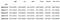Sample of the data

Visually show the stock price.

`# Visually Show The Stock Price(s)#Create and plot the graphplt.figure(figsize=(12.2,4.5)) #width = 12.2in, height = 4.5plt.plot( df['Close'],  label='Close')#plt.plot( X-Axis , Y-Axis, line_width, alpha_for_blending,  label)plt.xticks(rotation=45) plt.title('Close Price History')plt.xlabel('Date',fontsize=18)plt.ylabel('Price USD (\$)',fontsize=18)plt.show()`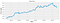The Close Price History of Amazon Inc.

Next, calculate the OBV.

`#Calculate the On Balance VolumeOBV = []OBV.append(0)for i in range(1, len(df.Close)):    if df.Close[i] > df.Close[i-1]: #If the closing price is above the prior close price           OBV.append(OBV[-1] + df.Volume[i]) #then: Current OBV = Previous OBV + Current Volume    elif df.Close[i] < df.Close[i-1]:          OBV.append( OBV[-1] - df.Volume[i])    else:          OBV.append(OBV[-1])`

Store the OBV into a new column and calculate the OBV exponential moving average, also store it into a new column as well. Then show the data.

`#Store the OBV and OBV EMA into new columnsdf['OBV'] = OBVdf['OBV_EMA'] = df['OBV'].ewm(com=20).mean()#Show the datadf`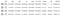Sample of the data set showing the two new columns ‘OBV’ & ‘OBV_EMA’

Plot the OBV and the OBV_EMA columns.

`#Create and plot the graphplt.figure(figsize=(12.2,4.5)) #width = 12.2in, height = 4.5#plt.plot( df['Close'],  label='Close')#plt.plot( X-Axis , Y-Axis, line_width, alpha_for_blending,  label)plt.plot( df['OBV'],  label='OBV', color= 'orange')plt.plot( df['OBV_EMA'],  label='OBV_EMA', color= 'purple')plt.xticks(rotation=45) plt.title('OBV/OBV_EMA')plt.xlabel('Date',fontsize=18)plt.ylabel('Price USD (\$)',fontsize=18)plt.show()`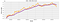A Plot Showing the OBV and OBV_EMA for Amazon visually

Create a function to signal when to buy and sell the asset.

`#Create a function to signal when to buy and sell an asset#If OBV > OBV_EMA Then Buy#If OBV < OBV_EMA Then Sell#Else Do nothingdef buy_sell(signal, col1, col2):  sigPriceBuy = []  sigPriceSell = []  flag = -1 #A flag for the trend upward/downward  #Loop through the length of the data set  for i in range(0,len(signal)):    #if OBV > OBV_EMA  and flag != 1 then buy else sell      if signal[col1][i] > signal[col2][i] and flag != 1:          sigPriceBuy.append(signal['Close'][i])          sigPriceSell.append(np.nan)          flag = 1      #else  if OBV < OBV_EMA  and flag != 0 then sell else buy      elif signal[col1][i] < signal[col2][i] and flag != 0:              sigPriceSell.append(signal['Close'][i])          sigPriceBuy.append(np.nan)          flag = 0      #else   OBV == OBV_EMA  so append NaN       else:         sigPriceBuy.append(np.nan)        sigPriceSell.append(np.nan)    return (sigPriceBuy, sigPriceSell)`

Create columns to store the buy and sell signals.

`#Create buy and sell columnsx = buy_sell(df, 'OBV','OBV_EMA' )df['Buy_Signal_Price'] = xdf['Sell_Signal_Price'] = x#Show the data framedf`

Show and plot the buy and sell signals for the stock.

`# Visually Show The Stock buy and sell signals#Create and plot the graphplt.figure(figsize=(12.2,4.5)) #width = 12.2in, height = 4.5plt.scatter(df.index, df['Buy_Signal_Price'], color = 'green', label='Buy Signal', marker = '^', alpha = 1)plt.scatter(df.index, df['Sell_Signal_Price'], color = 'red', label='Sell Signal', marker = 'v', alpha = 1)plt.plot( df['Close'],  label='Close Price', alpha = 0.35)#plt.plot( X-Axis , Y-Axis, line_width, alpha_for_blending,  label)plt.xticks(rotation=45)plt.title('The Stock Buy / Sell Signals')plt.xlabel('Date',fontsize=18)plt.ylabel('Close Price USD (\$)',fontsize=18)plt.legend( loc='upper left')plt.show()`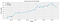A Plot Showing the Buy and Sell Signals for Amazon

So, it looks like the strategy does well for this data set, meaning you would’ve profited within this time period using this strategy for this data set, but remember that this indicator is not perfect and the strategy doesn’t guarantee success. A lot more testing is needed to be done before using this strategy and you may want to use other indicators with this strategy for more information on when to buy and sell the stock.

If you want to start an investment portfolio, then sign up with WeBull using this link and get FREE stocks just for opening an account and funding it with an initial deposit of \$100 or more! It’s free stocks that you can either sell, play with or create your own trading strategy with. For a free stock trading app, I highly recommend it.

Thanks for reading this article, and I hope you all learned something fun, new and interesting !

A programmer that loves Computer Science: https://www.youtube.com/user/randerson112358 https://www.youtube.com/channel/UCbmb5IoBtHZTpYZCDBOC1